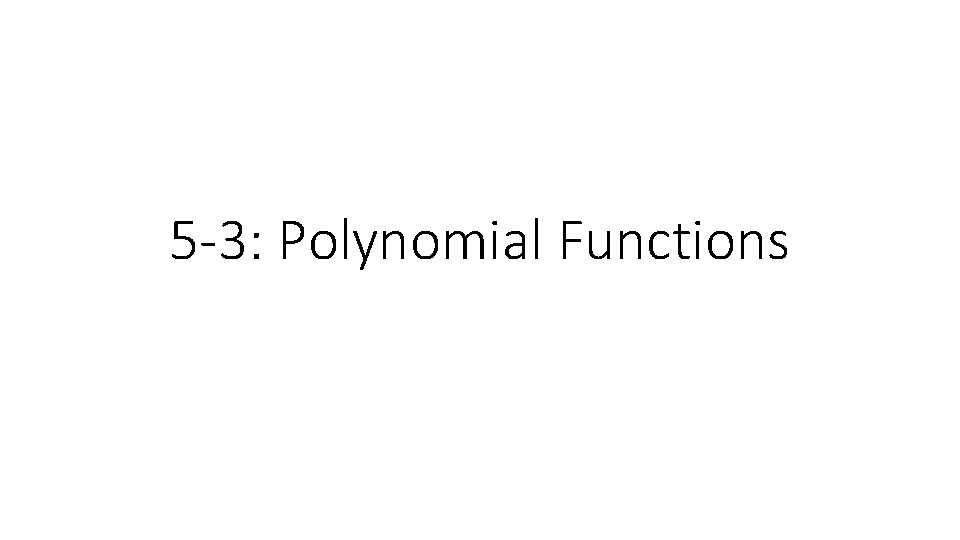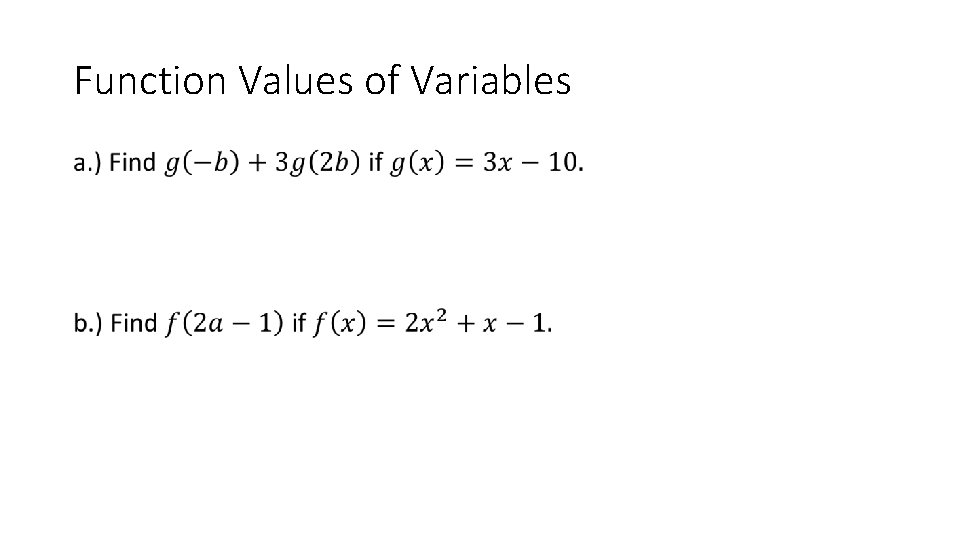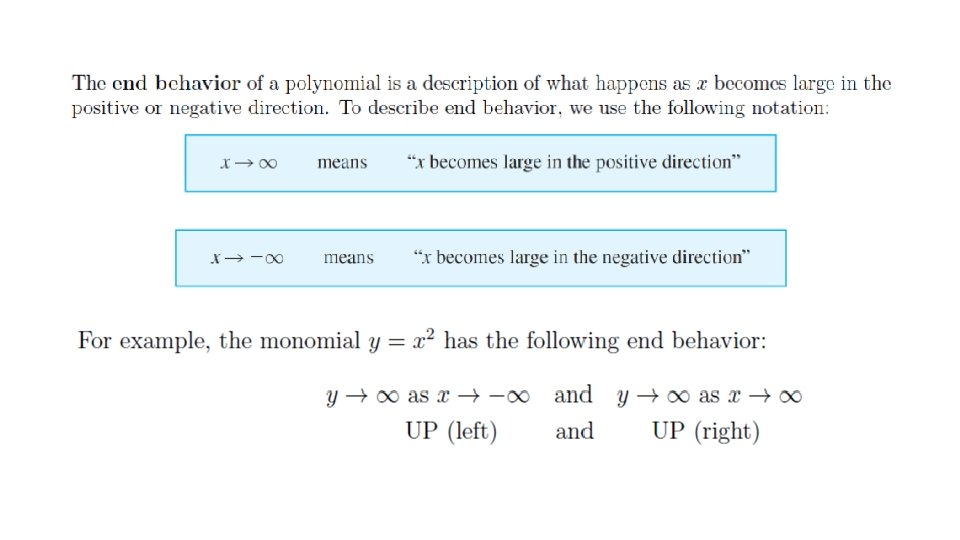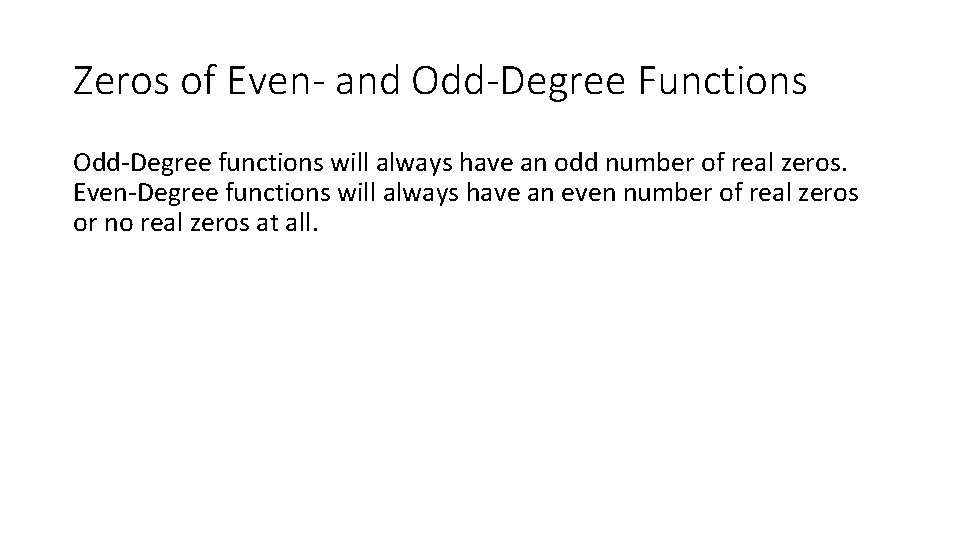# 5 3 Polynomial Functions A polynomial function is

• Slides: 115 -3: Polynomial FunctionsA polynomial function is a function of the form f (x) = a a n x nn + an – 1 x nn–– 11 +· · ·+ a 1 x + a 0 0 Where an 0 and the exponents are all whole numbers. leading coefficient For this polynomial function, ann is the leading coefficient, n is the degree. constant term degree aa 00 is the constant term, and n A polynomial function is in standard form if its terms are written in descending order of exponents from left to right.You are already familiar with some types of polynomial functions. Here is a summary of common types of polynomial functions. Degree Type 0 Constant 1 Linear 2 Quadratic 3 Cubic 4 Quartic Standard Form f (x) = a 0 f (x) = a 1 x + a 0 f (x) = a 2 x 2 + a 1 x + a 0 f (x) = a 3 x 3 + a 2 x 2 + a 1 x + a 0 f (x) = a 4 x 4 + a 3 x 3 + a 2 x 2 + a 1 x + a 0One Variable Polynomial, Degrees and Leading Coefficients •Function Values of Variables •Zeros of Even- and Odd-Degree Functions Odd-Degree functions will always have an odd number of real zeros. Even-Degree functions will always have an even number of real zeros or no real zeros at all.For the graph, • Describe the end behavior, • Determine if it’s Odd or Even-degree • State the number of zeros.Assignment Pg. 326 -327 #17 -39 odd